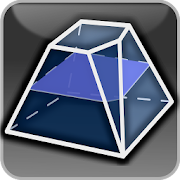## Geometryx: Geometry - Calculator helps you practice Grade HS Math - Lumos Educational App Store4.71
Price -fREE
\$0

#### DESCRIPTION:

Geometryx is an application that allows you to calculate quickly and easily the most important values and parameters of plane and solid figures and shapes. The application calculates an area, perimeter, circumference, diagonal length, volume, coordinates of the geometric centroid, height, side lenght, angle (acute, right, obtuse, straight, reflex), radius (inner, outer), edges, arc length, line segments, base area, lateral surface area and total surface area of three-dimensional geometric shapes. Geometryx is a simple calculator using trigonometric functions, Pythagorean theorem and Thales

#### OVERVIEW:

Geometryx: Geometry - Calculator is a free educational mobile app By .It helps students in grades HS practice the following standards HSG.CO.A.3.

This page not only allows students and teachers download Geometryx: Geometry - Calculator but also find engaging Sample Questions, Videos, Pins, Worksheets, Books related to the following topics.

1. HSG.CO.A.3 : Given a rectangle, parallelogram, trapezoid, or regular polygon, describe the rotations and reflections that carry it onto itself..

HS

#### STANDARDS:

HSG.CO.A.3

Developer:

Software Version: 2.2

Category:

### RELATED APPSEdSearch WebSearch